## Monday, May 5, 2014

### Review: Canon F-792SGA Scientific Calculator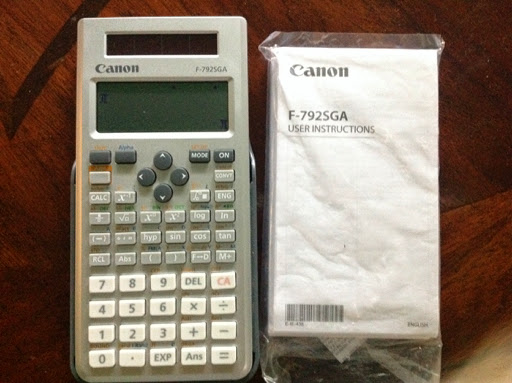Here it is, a full in-depth review of the Canon Eco-Friendly F-792SGA calculator. For a hilarious intro video to the calculator, just click here:

http://youtu.be/F4QJZSLYLyk

Quick Stats
Company: Canon
Price: The price ranges from \$13 to \$20.
Power: Solar, with battery backup (one CR2032 battery)
Where to Buy: Online. I am not aware of any stores in the United States (maybe Fry's?) that have them on sale. I got mine from amazon.com.
Package: Blister package. The calculator comes with two foldable manual sheets: one in English, the other in Spanish.
Special Note: The calculator's shell is made of recycled plastic.
Year Manufactured: first manufactured in 2013

Official web page from Canon: http://www.usa.canon.com/cusa/consumer/products/calculators/compact_calculators/f_792sga

At the time of this blog post, Canon does not offer an electronic version of the manual. Hopefully it will be uploaded soon.

Calculator Modes Offered
(First page)
1: COMP - Regular Calculator Mode
2: CMLX - Complex Number Mode
3: STAT - Statistics Mode
4: BASE - Base Calculations
5: EQN - Simultaneous Equations up to 4 x 4 systems, Quadratic, Cubic, and Quartic polynomial solver
6: TABLE - Function table of one variable
7: MATX - Matrices with operations for up to 4 x 4 matrices
8: VCTR - Vectors with operations for up to 3-element vectors
(Second Page)
1: INEQ - Inequality Solver
2: RATIO - Ratio Solver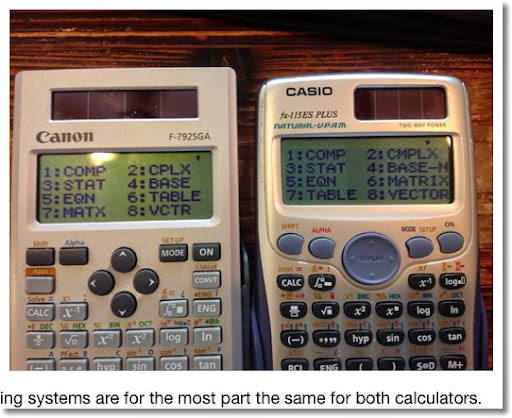General Math Mode

The F-792SGA allows for linear or textbook input and output. In textbook input, exact roots and multiples of π are displayed. Pressing the F-D key converts answers between exact and approximate.

Keyboard and the Apps Key

The keys are nice and responsive. Also, the keys stay in place when pressed and don't make slight shifts, giving the keyboard stability.

The keyboard is also nicely organized. The dedicated Apps button gives the user access to functions depending on the mode.

COMP: Π (product), Σ (sums), Max (of at least 2 numbers), Min (of at least 2 numbers), Q...r (quotient and remainder), Mod, LCM (of at least 2 integers), GCD (of at least 2 integers)

CMPLX: Polar and rectangular conversions, real part, imaginary part, conjugate, argument

STAT: ability to change regression type, edit data, regression variables, normal distribution area calculations

BASE: AND, OR, XOR, XNOR, NOT, NEG, base markers

EQN: change equation type

MATX: edit matrices, determinant, transpose, generate identity matrices, inverse of matrices (Inv), adjoint to matrices

VCTR: edit vectors, dot product. (Use × for cross product)

RATIO and INEQ: change type

In COMP mode, you can calculate numerical derivatives of f(X), definite integrals of f(X), random numbers, and random integers (Press [Alpha], [ . ]).

Solving Equations in COMP Mode

To solve an equation, type in the equation, follow it with a comma (Press [Shift], [ ) ]). Then press [Shift], [CALC]. You can use the variables A through F, X, Y, and M.

38 Built In Formulas

Canon has a nice feature that is exclusive to the Canon scientific calculator line: that is the 38 built in formulas. On the F-792SGA, just press [Alpha], [ ( ]. Then scroll to get the formula desired, press the equals key. You are prompted for the variables and the result is returned.

For reference, I will have the 38 formulas listed at the end of this review after the Verdict section.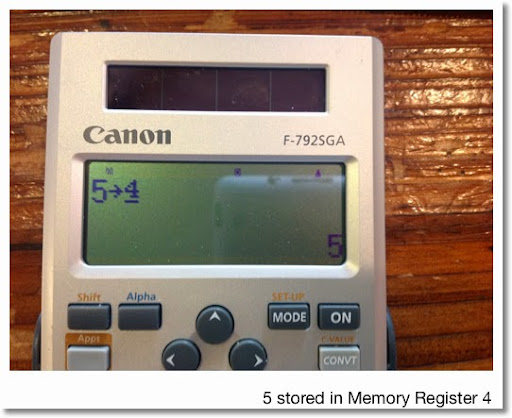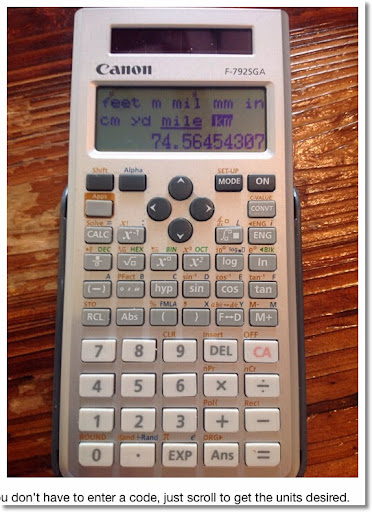Complex Mode

The complex mode is a separate mode. The nice thing is that there are real and imag functions. Unfortunately, transcendental functions (powers, logs, trig) are not available with complex numbers.

Statistics Mode

You can turn the frequency column on and off. The F-792SGA can store up to 80 single points or 40 pairs. With the frequency column turned on, the number of slots are halved.

Regressions available are: Linear, Exponential (y = a*e^(b*x) and y = a*x^b), Power, Logarithmic, Inverse, and Quadratic (nice!)

F-792SGA vs. Other Scientific Calculators

The display and operating system of the Canon F-792SGA is practically the same of Casio's current Natural-V.P.A.M calculators (such as the fx-115ES PLUS). However, this is not a straight-knock off of the Casio counterpart.

Here are some differences:

* The F-792SGA has 79 scientific constants and 170 conversion pairs compared to the fx-115ES PLUS's 40 and 40, respectively. For further comparison purposes, the Sharp EL-W516X has 52 and 44, respectively. The nice thing about the F-792SGA is that you don't need to type a code to access the constants and conversions.

* The F-792SGA has a ratio solving mode, which I think is unique to this calculator (or to the Canon line?). This mode solves for X in two ratio statements: a:b=X:d and a:b=c:X. This is in place of the fx-115ES PLUS' verify mode.

* Up to 4 x 4 matrices can be used with the F-792SGA. This is on par with the Sharp EL-W516X. However, I like the matrix handling and operation on the F-792SGA better.

* The F-792SGA is one of the few solar scientific calculators to solve quartic equations, if not the only one.

* The F-792SGA has 19 memory registers: A-F, X, Y, M, and 0-9. The numeric storage registers are good for longer-term storage of constants. This is first calculator of this time to allow the use of numeric registers.

* The F-792SGA has 38 built in formulas, exclusive to Canon scientific calculators. This is referring to all non-graphing calculators.

Verdict

The Canon F-792SGA is a solid calculator and can rival the solar scientific calculators that are available. Again, you may not be able to find Canon calculators in most stores (that is unfortunate), so the most realistic way to get one is to order online (assuming it is available in your country).

I hope you enjoyed this review.

Eddie

List of Formulas included with the F-792SGA

1. Area of a Triangle: S = 1/2 * b * c * sin A

2. Area of a Circle: S = π * r^2

3. Fan-Shaped Area (Sector): S = 1/2 * r^2 * θ

4. Area of a Parallelogram: S = a * b * sin θ

5. Area of an Ellipse: S = π * a * b

6. Area of a Trapezoid: S = 1/2 * (a + b) * h

7. Surface Area-Sphere: S = 4 * π * r^2

8. Surface Area-Cylinder: S = 2 * π * r * (h + r)

9. Volume-Sphere: S = 4/3 * π * r^3

10. Volume-Cylinder: V = π * r^2 * h

11. Volume-Cone: V = 1/3 * π * r^2 * h

12. Sum-Arithmetic Progression: S = 1/2 * n * (2*a_0 + (n-1)*d))

13. Sum-Geometric Progression: S = a_0 * (r^n - 1)/(r - 1)

14. Σ(n^2) = 1/6 * n * (n + 1) * (2*n + 1)

15. Σ(n^3) = (1/2 * n * (n + 1))^2

16. Distance between two points: √((x_2 - x_1)^2 + (y_2 - y_1)^2)

17. Included angle between two lines: θ = atan((k_2 - k_1)/(1 + k_1 * k_2))

18. Law of Cosines-find side: a = √(b^2 + c^2 - 2*b*c*sin A)

19. Law of Sines-find side: a = 2 * r * sin A

20. Distance: d = v_0 * t + 1/2 * a * t^2

21. Velocity: v = v_0 + a * t

22. Circular Motion-Finding Period with velocity: T = 2 * π * r/v

23. Circular Motion-Finding Period with angular velocity: T = 2 * π/omega

24. Period of Simple Pendulum: T = 2 * π * √(l/g)

25. Electric Oscillation Frequency: f = 1/(2 * π * √(L * C))

26. Resistance: R = ρ * l/s

27. Joule's Theorem: P = V^2/R

28. Joule's Theorem: P = I^2 * R

29. Shunt Resistance: R = (R_1 * R_2)/(R_1 + R_2)

30. Kinetic Energy: E = 1/2 * m * v^2

31. Gravitational Potential Energy: E = m * g * h

32. Centrifugal Force: F = m * v^2/r

33. Centrifugal Force: F = m * omega^2 * r

34. Law of Gravity: F = G * M * m/r^2 (G = 6.6728*10^-11)

35. Electric Field Intensity: E = Q/(4 * π * epsilon * r^2)

36. Heron's Formula: S = √(Φ*(Φ-a)*(Φ-b)*(Φ-c)) where Φ=(a+b+c)/2. Only a,b, and c are asked for.

37. Optics-Reflective Index: E = sin I/sin r

38. Optics-Critical Angle-Total Reflection: θ = asin(n_2/n_1)

** g is assumed to be g = 9.80665 m/s^2. Use SI units.

Note: These formulas are included with most Canon scientific calculators, like the F-710. The notable exception is the F-604, where the formulas are not present.
Source: Canon F-792SGA Manual

This blog is property of Edward Shore. 2014

1.I need to know; are old formulas in the replay memory LOST when the Canon F-792SGA times out? The FX-115MS does this, --unless I press a key periodically to keep the Casio on.
/Lonewolf™

1.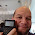Lonewolf,

Sorry for the late reply. To find out, I have put a couple of formulas and let the calculator time out.

Yes, the formulas are lost after the F-792SGA times out.

Eddie

2.Thanks Eddie. . .
/Lonewolf™

2.Thank you so much for posting. I have been looking for something like this.solar panels for petrol station

1.Malik,

You are welcome. Glad to do it.

Eddie

3.This calculator seems to be an extension of the Casio engine, as if they licensed it. Its similar to the HP300s, which has even more Casio feel to it. The give away to the HP300s is the way the LCD appears, the formation of the aphanumerics, the annunciators on the LCD, the keyboard layout, the execution sequences of the firmware, being more similar to Casio versus the very different response of TI36X Pro.

4.This comment has been removed by the author.

5.Is the Canon F-792SGA actually based on the Casio FX-115ES Plus / FX-991ES Plus C? Or is the Canon based on the older NON-"Plus" models?...The reason why I'm asking is because I thought the Canon was pretty much a Casio FX-115ES Plus / FX-991ES Plus C clone, but only better...However, I have just found out that there are things that the Casio FX-115ES Plus / FX-991ES Plus C does that the Canon apparently DOES NOT do, such as the following:

DISTRIBUTION
normal, inverse normal, binomial, Poisson

POLYNOMIAL EQUATIONS
calculates vertex of parabola (S functions can be programmed)

SYSTEMS OF EQUATIONS
Infinite Solution & No Solution enunciators

FUNCTION TABLE
2 functions, f(x) & g(x) calculated side-by-side
(Canon - 1 function, f(x))

MATRIX
ref & reduced-ref

UNIQUE FEATURES
VERIFY checks results of number & algebra expressions
PreAns (PreAns + Ans generates Fibonacci Sequence)
recurring decimal (gives ALL 60 digits of 1/61)
remainder division (N,T have mod function)
old FX-115MS SOLVE did not require comma-variable to select
old FX-115MS % could calculate percent + or - & percent change
old FX-115MS could COPY multi-statements

So it looks like the Canon isn't simply a Casio FX-115ES Plus / FX-991ES Plus C but better, it looks like the Canon isn't able to do some of the stuff that the Casio can do...By the way, I got the above from an Amazon review...here is the full list from the reviewer -->

http://www.amazon.com/review/R2NXOSQYFO1Q8H/ref=cm_cr_rdp_perm?ie=UTF8&ASIN=B009FFPEH0

Can you please check it out and tell me what you think??...

I'm going crazy trying to decide. I'm taking grade 11 & 12 pre-calculus, chemistry, and physics...and will be moving on to a 4-year physics/space science university degree.

1.Vince,

I would say that the Canon F-792SGA is closer (if not surpasses) to the fx-115E PLUS. However, the F-792SGA has a larger constant library, conversion library, and a formula library. If those three features are important to you, I would go for the F-792SGA.

2.Sweet, I'm going to be getting the Canon most likely.

One more question...it looks like the Canon can only do 1 function for a table while the Casio can do two. Is this something that a pre-calculus 11 and 12 student will be missing out from? Do pre-calculus 11 and 12 students even come across dual-function graphs, or is that more university stuff (in which case, I'll probably be allowed a graphing calculator by then, but not at the moment)...

3.Vince,

Going by experience, I do not remember doing dual-graphs in pre-calculus. However, this was 20 years ago. You should be OK.

If you want to be sure, check out the current pre-calc textbooks - which you can find at local and university libraries. Or, the bookstore has pre-calc review books that covers the majority of the topics.

Eddie

6.Just stay with us and enjoy the professional solutions that we give to your backup, survellance and network needs.
Surveillance And Security Systems in Nigeria

7.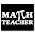Very knowledgeable post. This will help to solve various scientific problems but I was looking for online math tutor for solving trignomatric problems.

8.This comment has been removed by the author.

9.i want to buy new calculator for college use in geomatic course and thinking buying Canon F-718. Is it compatible?
may i ask you opinion which one is better ?
1.Canon , F-718S
2.Canon F-789SGA
3.Casio fx-570

1.I lean towards choices 2 and 3.

10.I would like to buy a new calculator. I'm an engineering student and I would like a calculator I could use until my licensure exams and even until I'm an engineer. Would you recommend this or the Casio 991 EX PLUS Classwiz?

11.Hello sir, Can you please tell me if Canon f-789sga has bult in formulas like area of triangle?

12.Nice blog it is informative thank you for sharing Tableau Online Training

13.is this programmable or non programmable

14.I've proper selected to build a blog, which I hold been deficient to do for a during. Acknowledges for this inform, it's really serviceable! 175 lbs to kg

### Casio fx-5800P and TI-84 Plus CE: Simplify Radicals and August TI-95 ProCalc Month

Casio fx-5800P and TI-84 Plus CE: Simplify Radicals and August TI-95 ProCalc Month Introduction The program SIMPRAD simplifies ra...